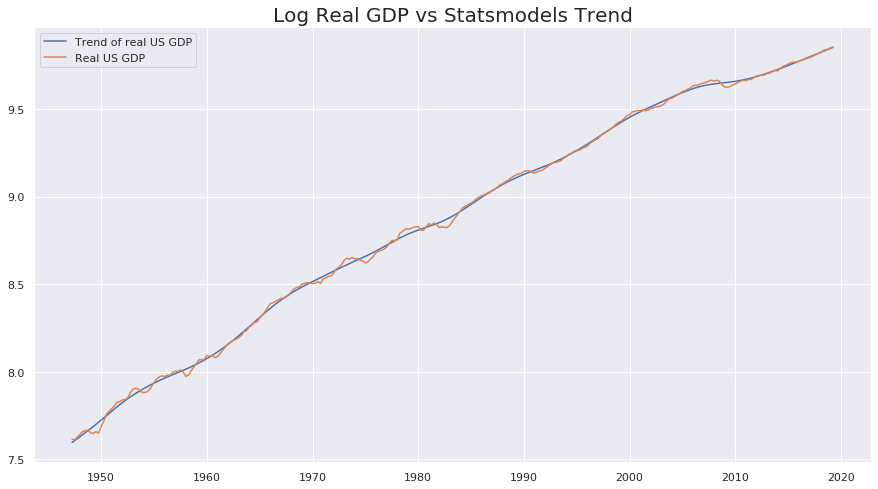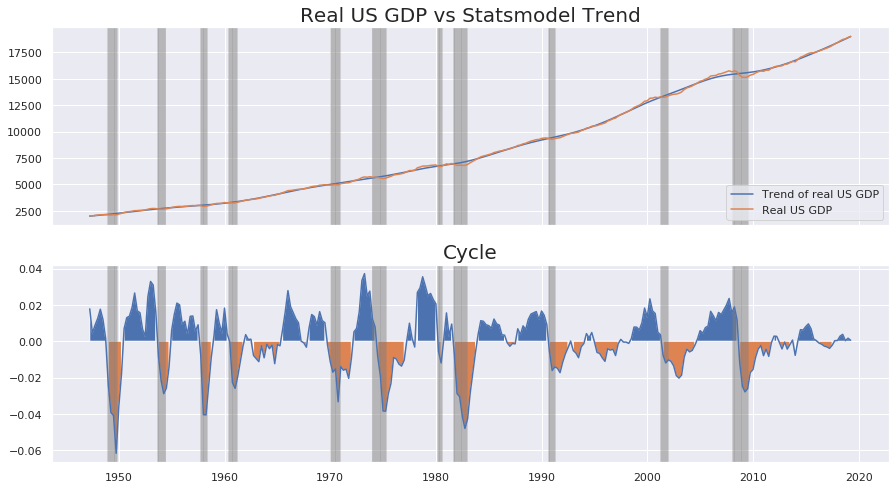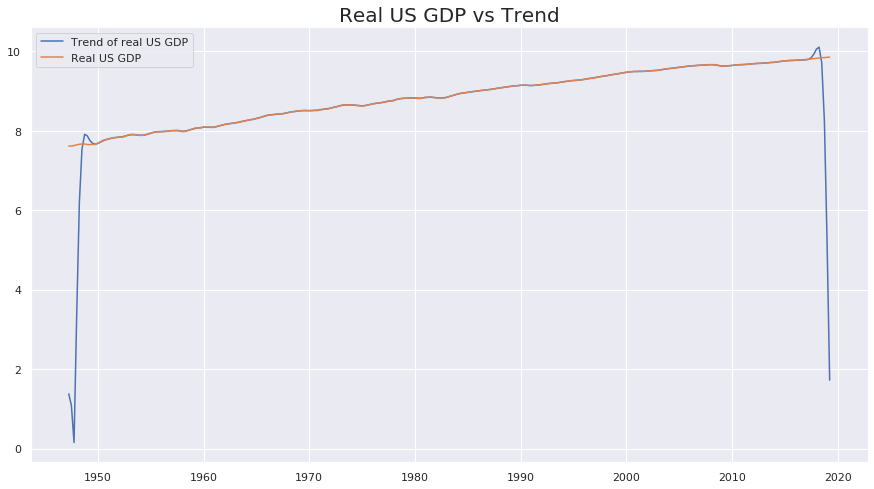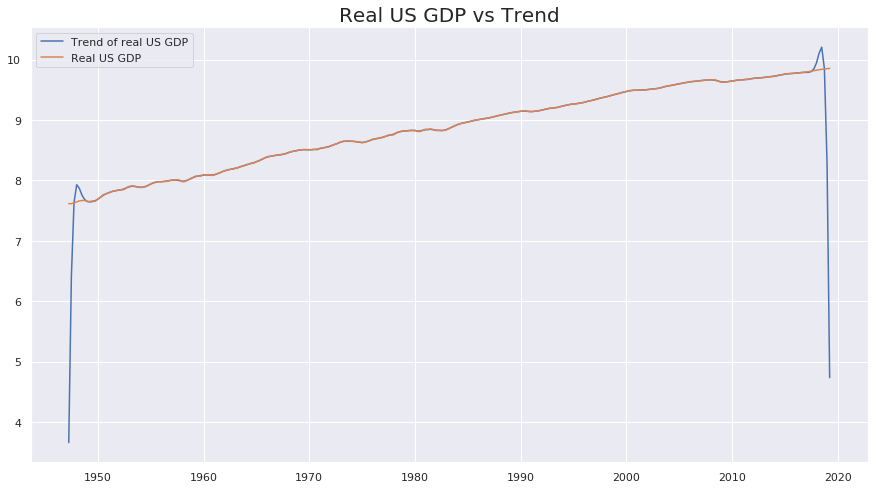In :
import pandas as pd
import numpy as np
import matplotlib.pyplot as plt

import statsmodels.api as sm
from scipy.optimize import minimize, show_options

import seaborn as sns
sns.set()


# Introduction - HP Filters¶

In this notebook, I analyze the Hodrick Prescott filter (HP filter) to detrend macroeconomic time series in three different ways:

• First, I use the Statsmodel implementation of the HP filter
• Then, I compute an exact solution from partial derivatives
• Finally, I use Scipy's minimize function to obtain a numeric solution

I use the filter on the US real GDP time serie provided by the Federal Reserve as an illustration (the time serie can be dowloaded from the FRED website).

# Data Retrieval and Pre-Processing¶

Data are stored as a Pandas DataFrame using the read_csv method. The keep_date value below allows to limit the analysis to data posterior to a given date.

I then use a Numpy array to store the log real GDP time serie.

In :
real_gdp = pd.read_csv('real_gdp_us.csv',index_col=0)
real_gdp.index = pd.to_datetime(real_gdp.index)

#Keep date from:
keep_date = pd.to_datetime('1947-01-01')

real_gdp = real_gdp[real_gdp.index > pd.to_datetime(keep_date)]

log_real_gdp = np.log(real_gdp['GDPC1']).values
log_real_gdp2 = log_real_gdp[1:len(log_real_gdp)-1]

date_index = real_gdp.index

rec = pd.read_csv('USREC.csv',index_col = 0) #US Recessions, monthly
rec.index = pd.to_datetime(rec.index)
#rec = rec[rec == 1]
rec = rec[rec.index > "1947-01-01"]
rec = rec[rec['USREC'] == 1]


# Mathematical Analysis¶

The HP filter allows to obtain the trend by minimizing the following objective function:

$$\underset{\{\tau_t | t\in n\}}{min} f(\tau_t) = \sum_{t=0}^{n}\left( Y_t - \tau_t \right)^2 - \lambda * \sum_{t=1}^{n-1}\left( (\tau_{t+1} - \tau_t) - (\tau_t - \tau_{t-1}) \right)^2$$

Where $Y_t$ is the original time serie and $\lambda$ a parameter. Hodrick and Prescott advocate a $\lambda$ value of 1,600 for quarter series such as the log real GDP I am using as an illustration.

The Jacobian of the objective function is:

$$J_f: \begin{cases} R^n \rightarrow R^n \\ (\tau_t)_{t \in n} \rightarrow (\frac{\partial f}{\partial \tau_t})_{t \in n} \end{cases}$$

We have $\forall t \in [2,n-2]$: $$\frac{\partial f}{\partial \tau_t} = 2 * \left(\tau_t - Y_t + \lambda * \left( \tau_{t+2} - 4 \tau_{t+1} + 6 \tau_{t} - 4 \tau_{t-1} + \tau_{t-2} \right) \right)$$

Some algorithm may also require the Hessian of the objective function. It is trivial to show from the Jacobian that: $$\begin{cases} \frac{\partial^2 f}{\partial^2 \tau_t} = 2 * (1 + 6 * \lambda) \\ \frac{\partial^2 f}{\partial \tau_t \partial \tau_{t+2}} = 2 * \lambda \\ \frac{\partial^2 f}{\partial \tau_t \partial \tau_{t-2}} = 2 * \lambda \\ \frac{\partial^2 f}{\partial \tau_t \partial \tau_{t+1}} = - 8 * \lambda \\ \frac{\partial^2 f}{\partial \tau_t \partial \tau_{t-1}} = - 8 * \lambda \end{cases}$$ All other partial derivatives are null.

For the remainder, we fix the value of $\lambda$ to 1600.

In :
l = 1 #lambda is reserved keyword in Python


# Statsmodels' Implementation¶

The Statsmodels library provide a ready made implementation. This version corrects a certain number of issues with the standard implementation. As we will see later, a direct implementation of the HP filters lead to end-points bias which needs to be removed from the analysis.

We plot below the log real GDP vs the trend returned by Statsmodels.

In :
# Store the cycle and the trend
cycle, trend = sm.tsa.filters.hpfilter(log_real_gdp, 1600)

fig, ax = plt.subplots(1, 1,sharex=True,figsize=(15, 8))

df_trend = pd.DataFrame(index = real_gdp.index, data = trend)
df_log_real_gdp = pd.DataFrame(index = real_gdp.index, data = log_real_gdp)

ax.set_title('Log Real GDP vs Statsmodels Trend',size = 20);

ax.plot(df_trend);
ax.plot(df_log_real_gdp);
ax.legend(['Trend of real US GDP','Real US GDP']);We can now analyze the cycle.

I first plot the real US GDP vs its trend returned by Statsmondel and then the cycle. Statsmodel's GitHub page shows that the cycle has been implemented as

cycle = real data - cycle

Therefore, we would obtain the same result by taking the difference between the real_gdp and trend variables.

Please note that the grey areas represent NBER US recessions.

In :
fig,ax = plt.subplots(2,1,sharex = True, figsize = (15,8))

df_trend = pd.Series(index = real_gdp.index, data = np.exp(trend))
df_cycle = pd.Series(index = real_gdp.index, data = cycle)

zero = [0 for t in trend]

ax.plot(df_trend);
ax.plot(real_gdp);
ax.legend(['Trend of real US GDP','Real US GDP']);
ax.plot(df_cycle)
ax.fill_between(real_gdp.index,zero,df_cycle,where=df_cycle > 0);
ax.fill_between(real_gdp.index,zero,df_cycle,where=df_cycle < 0);

ax.set_title('Real US GDP vs Statsmodel Trend',size = 20)
ax.set_title('Cycle',size = 20);

for d in rec.index:
ax.axvspan(d, d + pd.DateOffset(months=1), alpha=0.2, color='grey')
ax.axvspan(d, d + pd.DateOffset(months=1), alpha=0.2, color='grey')As we can see from the graphs above and one could expect, NBER recessions coincide with negative peaks in the HP filter. This concludes the analysis of the Statsmodels implementation of the HP filter.

# Exact Solution Implementation¶

We now turn to the implementation of an exact solution. Using the Jacobian previously computed, we know that the objective function is minimized when:

$$\forall \tau_t, \frac{\partial f}{\partial \tau_t} = 0$$

This can be reduced to solving:

$$A * \tau = Y$$

This a system of equation with $n$ unknown and $n$ independant equations. It can therefore be solved.

Where $A$ is a matrix for which the coefficients are determined from the Jacobian, $\tau$ is the vector of solutions and $Y$ is the observed time serie. I defer the computation of the coefficient of the matrix $A$ to this article.

The implementation in Python is available below:

In :
card = len(log_real_gdp)

A = np.eye(card) * (1 + 6 * l)
for i in range(card):
if i < card - 1:
A[i,i+1] = -4 * l
A[i+1,i] = -4 * l
if i < card - 2:
A[i,i+2] = l
A[i+2,i] = l
if i == 2 or i == card - 2:
A[i,i+1] = l
A[i+1,i] = l

A[0,1] = -2
A[1,0] = -2
A[card-1,card-2] = -2
A[card-2,card-1] = -2

I = np.zeros((card,card))

tau = np.linalg.inv(A.T.dot(A) + I.T.dot(I)).dot(A.T).dot(log_real_gdp)


I now plot the trend computed above vs the log real US GDP.

In :
fig, ax = plt.subplots(1, 1,sharex=True,figsize=(15,8))

df_tau = pd.Series(index = real_gdp.index, data = tau)

ax.plot(df_tau)
ax.plot(df_log_real_gdp)
ax.legend(['Trend of real US GDP','Real US GDP']);
ax.set_title('Real US GDP vs Trend',fontsize=20);This graph illustrates the issue of a direct implementation of the HP filter. End-points (i.e. data for the first and last years of the computation) are stuck to value close to 0. There is no need to study the behaviour of this solution further as the endpoint will be highly biased.

The use of Statsmodel is therefore highly superior to a direct implementation.

# Scipy Optimization Method¶

The last method used is a minimization of the function using Scipy's optimize module. This requires providing to Python an explicit objective function, its Jacobian and its Hessian. These steps are describe below.

Since the logic behind the solution is similar to the direct implementation, we should expect the end-points bias to occur again.

In :
def obj_fun(tau):
"""
Objective function of the HP filter
"""
first_part = np.power(log_real_gdp - tau,2)

tau_plus_1 = np.append(tau[1:len(tau)],)
tau_minus_1 = np.append(,tau[0:len(tau)-1])
second_part = (tau_plus_1 - tau) - (tau - tau_minus_1)
second_part = np.power(second_part,2)
return (np.sum(first_part) - l * np.sum(second_part))

def jacobian(tau):
"""
Jacobian of the objective function
"""
tau_plus_2 = np.append(tau[2:len(tau)],[0,0])
tau_plus_1 = np.append(tau[1:len(tau)],)
tau_minus_1 = np.append(,tau[0:len(tau)-1])
tau_minus_2 = np.append([0,0],tau[0:len(tau)-2])

return 2*(tau -log_real_gdp + l * (tau_plus_2 - 4 * tau_plus_1 + 6 * tau - 4 * tau_minus_1 + tau_minus_2))

H_matrix = np.eye(len(log_real_gdp))*(2 *(1 + 6 * l))
for i in range(len(log_real_gdp)-2):
H_matrix[i,i+2] = 2 * l
H_matrix[i+2,i] = 2 * l

for i in range(len(log_real_gdp)-1):
H_matrix[i,i+1] = -8 * l
H_matrix[i+1,i] = -8 * l

def hessian(tau):
"""
Some method of Optimize.minimize expect a Jacobian matrix
"""
return H_matrix


# Computing the Trend¶

Having obtained the objective function and its jacobian, we can now run the optimization function from Scipy. The algorithm requires an initial solution, which we provide as a vector of 0. This is an arbitrary decision, which may sometimes impact the solution returned by the algorithm (or prevent the optimization to occur).

With the jacobian and hessian available, many methods are available. I chose the trust-exact method for optimization in the example below.

In :
tau_init = np.zeros(len(log_real_gdp))
res = minimize(obj_fun,
tau_init,
method='trust-exact',
jac = jacobian,
hess=hessian,
options={'disp':True})

Optimization terminated successfully.
Current function value: 34.944452
Iterations: 8
Function evaluations: 9
Hessian evaluations: 9


Once again, we can plot the log real US GDP vs the trend computed above.

In :
fig, ax = plt.subplots(1, 1,sharex=True,figsize=(15,8))

df_res_x = pd.DataFrame(index = real_gdp.index, data = res.x)

ax.plot(df_res_x)
ax.plot(df_log_real_gdp)
ax.legend(['Trend of real US GDP','Real US GDP']);
ax.set_title('Real US GDP vs Trend',fontsize=20);As expected, this method yields the same end-points bias.

# Conclusion¶

In this notebook, I implemeted an HP filter on US real GDP using three methodologies:

• Statsmodels' library
• Directly solving the linear system of partial derivatives
• Using Scipy's minimize function to obtain a numerical approximation

Of the three methodologies, only the first one overcome the issue of end-points bias.

The implementation of the third one is an interesting use case of Scipy's minimize with a very simple Hessian. It can be be useful to refer to this example for more complex issues directly related to the use Scipy's Hessian.